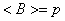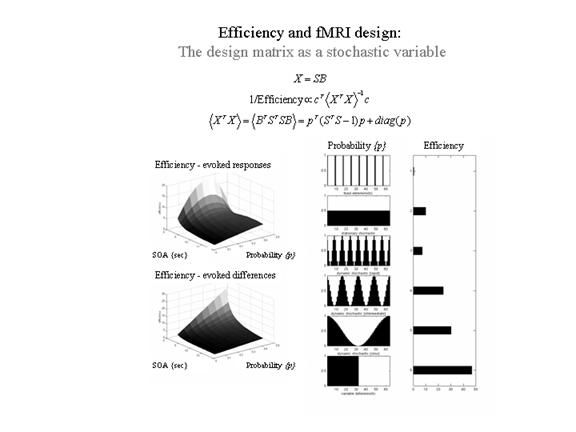Figure 12

Efficiency as a function of occurrence probabilities p. for a model X formed by post-multiplying S (a matrix containing n columns, modeling n possible event-related responses every SOA) by B. B is a random binary vector that determines whether the nth response is included in X or not, where. Right panels: A comparison of some common designs. A graphical representation of the occurrence probabilities p expressed as a function of time (seconds) is shown on the left and the corresponding efficiency is shown on the right. These results assume a minimum SOA of one second, a time-series of 64 seconds and a single trial-type. The expected number of events was 32 in all cases (apart from the first). Left panels: Efficiency in a stationary stochastic design with two event types both presented with probability p every SOA. The upper graph is for a contrast testing for the response evoked by one trial type and the lower graph is for a contrast testing for differential responses.